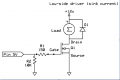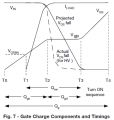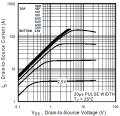# Mosfet switching times, switching losses and performance in linear vs saturation region.

#### reddydeepak

Joined Sep 19, 2017
7
I want to use a MOSFET (say IRLZ44n) to control 12V DC motor using Arduino's PWM. Since I posses limited knowledge of electronics I did some research on MOSFETs and need help to better understand it.1. I want to calculate switching time of MOSFET. By using equations like Q = CV and Q = It and assuming Arduino provides gate current of 20mA @5V, switching times can be found by looking at value of charges in datasheet. But is it really that simple. Based on the graph shown below, it seems like the turn on time depends on Vgs, which is changing with time. If this is true, can we use I = C*dv/dt to calculate turn on time? If yes, what will be value of capacitances?2. Since the mosfet is driven by Arduino's PWM how to ensure that charging and discharging time is less than PWM is switching frequency (say 490Hz operating at 50% duty cycle)? How to calculate switching losses and how can I minimize it?

3. Based on the graph shown below, saturation region is where current stops increasing. Also, according to wikipedia, MOSFET is in saturation region if Vds> (Vgs-Vth). How is this possible if Vds falls to zero when Vgs is applied? Also, When i look at the graph of Ids vs Vds, I cannot understand why Ids rises with Vds, when clearly it should as shown in Gate charge curve. Am i reading the graph of Ids vs Vds incorrectly? What am I missing?4. From the perspective of performance i.e. switching time and losses, when using PWM where should the operating point of MOSFET be? Linear/Ohmic or Saturation?

#### crutschow

Joined Mar 14, 2008
32,850
1. I want to calculate switching time of MOSFET. By using equations like Q = CV and Q = It and assuming Arduino provides gate current of 20mA @5V, switching times can be found by looking at value of charges in datasheet. But is it really that simple. Based on the graph shown below, it seems like the turn on time depends on Vgs, which is changing with time. If this is true, can we use I = C*dv/dt to calculate turn on time? If yes, what will be value of capacitances?
You use t = Q / I to calculate the worst-case turn-on time where Q is the total gate-charge given in the data sheet.
2. Since the mosfet is driven by Arduino's PWM how to ensure that charging and discharging time is less than PWM is switching frequency (say 490Hz operating at 50% duty cycle)? How to calculate switching losses and how can I minimize it?
The switching losses can be reasonably estimated by multiplying the switching frequency times the supply voltage times the load current times the rise/fall time.
3. Based on the graph shown below, saturation region is where current stops increasing. Also, according to wikipedia, MOSFET is in saturation region if Vds> (Vgs-Vth). How is this possible if Vds falls to zero when Vgs is applied?
Vds> (Vgs-Vth) is true in the saturation region.
Vds falls towards zero when more Vgs is supplied as it goes into the ohmic region.
I cannot understand why Ids rises with Vds,
Because it is in the ohmic region with a fixed Vgs.
4. From the perspective of performance i.e. switching time and losses, when using PWM where should the operating point of MOSFET be? Linear/Ohmic or Saturation?
For minimum loss you want the MOSFET to be fully-on in the ohmic region with Vds at a minimum.
The saturation region is of no interest when using the MOSFET as a switch.
The minimum Vgs to apply for this fully-on state is given in the MOSFET data sheet as the test condition for when the minimum Rds(on) value is measured.

•reddydeepak

#### reddydeepak

Joined Sep 19, 2017
7
You use t = Q / I to calculate the worst-case turn-on time where Q is the total gate-charge given in the data sheet.
Thank you for taking time to reply to this thread. I understand that considering worst case rise / fall time is best way to go. But how will this time fare against PWM frequency because charging and discharging times have to be less than PWM frequency . The reason I am asking this is to get a better estimation of losses and optimize it.

#### crutschow

Joined Mar 14, 2008
32,850
But how will this time fare against PWM frequency because charging and discharging times have to be less than PWM frequency .
That's why you drive the gate with a low impedance driver so that the rise and fall times are much less than the period of the PWM signal (ideally no more than a few percent).

#### reddydeepak

Joined Sep 19, 2017
7
That's why you drive the gate with a low impedance driver so that the rise and fall times are much less than the period of the PWM signal (ideally no more than a few percent).
Got it. Thank You for your time.

#### Janis59

Joined Aug 21, 2017
1,717
However, the =1/sum(Ton, Trise, Toff, Tfall)<<<F(T) if we speak on the auto-oscillation regime. I was very surprized when first met this effect.
For example, if I use an ixfh42n60 at Clapp circ, where this 32+23+60+17=112ns or expected F(max)=9 MHz works extremely well even on full power at 40 MHz, but some exemplars are able (with some not so nice thermic effects) be altered up to 105 MHz!!! That is observed with my own eyes otherhow I not be telling it. I have an feeling that C(gs) have far more serious impact on F(T).